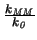Next: 6.3.1 Output Stage of Up: 6. Convergence and Damping Previous: 6.2.5 The New Method

# 6.3 Examples

The following small examples are aimed at proving the usefulness of one or the other approach. They have been selected for their simplicity and for their ability to represent typical or critical situations occurring in mixed-mode device simulations.

Interesting is a comparison of the convergence properties to the ideal case when the solution is used as an initial-guess for the node voltages and only the device equations need to be solved. With k0 being the number of iterations in this ideal case one can define a measure for the convergence by

 K =(6.18)

with kMM being the number of iterations for real mixed-mode simulation with vanishing initial-guess ( x = 0). K will be called the degradation factor in the following.

Mayaram and Pederson  stated that their simple circuits containing normally only one transistor converged within 8-9 iterations using the modified two-level Newton algorithm. As for each Newton step the device simulator has to be called which performs a Newton iteration itself, the total number of iterations is much larger. From a computational point of view the circuit iterations can be neglected. Assuming that the device simulator converges within 20 iterations for the first call and within 10 iterations for all consecutive calls gives a total of approximately 100 device simulator iterations. As this estimate is optimistic one can assume the modified full-Newton approach to be more efficient whenever convergence is obtained within less than 100 iterations since one iteration of the device simulator is approximately as expensive as one iteration of the complete equation system.Next: 6.3.1 Output Stage of Up: 6. Convergence and Damping Previous: 6.2.5 The New Method
Tibor Grasser
1999-05-31